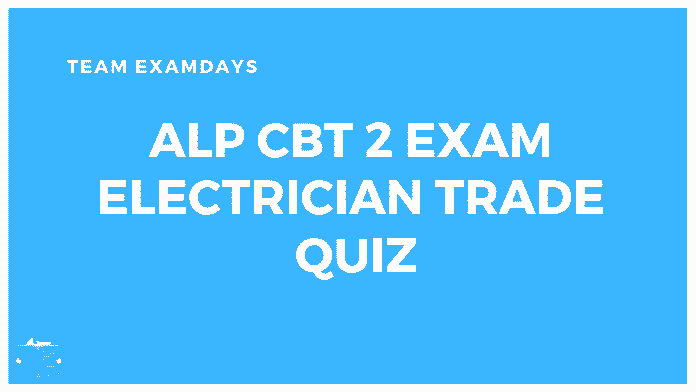ALP CBT 2 Electrician Trade Quiz

RRB ALP CBT 2 Electrician Trade questions MCQ Quiz which is helpful for ALP. RRB ALP CBT 2 Exam starts on 21 January onwards 2019. The maximum weight of ALP CBT 2 is General Science. you can download the Basic General Science and Engineering PDF. You can also download the ALP Practise Papers PDFYou can’t ignore the Trade Part

Question 1: One-newton meter is the same as.?

A.one watt
B.one joule
C.five joules
D.one joule second

one joule

Question 2: 6-ohm resistors are connected to form a triangle. What is the resistance between any two corners

A.3/2 Ohm
B.6 Ohm
C.4 Ohm
D.8/3 Ohm

4 Ohm

Question 3: Ohm’s law is not applicable to

A.semi-conductors
B.D.C. circuits
C.small resistors
D.high currents

semi-conductors

Question 4: Two copper conductors have equal length. The cross-sectional area of one conductor is four times that of the other. If the conductor having a smaller cross-sectional area has a resistance of 40 ohms the resistance of another conductor will be

A.160 ohms
B.80 ohms
C.20 ohms
D.10 ohms

10 ohms

Question 5: A nichrome wire used as a heater coil has the resistance of 2 ohms. For a heater of 1 kW at 200 V, the length of wire required will be

A.80 m
B.60 m
C.40 m
D.20 m

80 m

Question 6: Temperature coefficient of resistance is expressed in terms of

A.ohms/°C
B.mhos/ohm°C
C.ohms/ohm°C
D.mhos/°C

ohms/ohm°C

Question 7: Which of the following materials has the least resistivity ?

A.Zinc
C.Mercury
D.Copper

Copper

Question 8:When current flows through heater coil it glows but supply wiring does not glow because

A.current through supply line flows at slower speed
B.supply wiring is covered with in¬sulation layer
C.resistance of heater coil is more than the supply wires
D.supply wires are made of superior material

resistance of heater coil is more than the supply wires

Question 9:The condition for the validity under Ohm’s law is that

A.resistance must be uniform
B.current should be proportional to the size of the resistance
C.resistance must be wire wound type
D.temperature at positive end should be more than the temperature at negative end

resistance must be uniform

Question 10:Which of the following statement is correct ?

A.A semi-conductor is a material whose conductivity is same as be¬tween that of a
conductor and an insulator
B.A semi-conductor is a material which has conductivity having average value of conductivity of metal and insulator
C.A semi-conductor is one which con¬ducts only half of the applied voltage
D.A semi-conductor is a material made of alternate layers of conducting material and insulator

A semi-conductor is a material whose conductivity is same as be¬tween that of a
conductor and an insulator

Question 11:A rheostat differs from potentiometer in the respect that it

A.has lower wattage rating
B.has higher wattage rating
C.has large number of turns
D.offers large number of tapping

has higher wattage rating

Question 12:An open resistor, when checked with an ohm-meter reads

A.zero
B.infinite
C.high but within tolerance
D.low but not zero

infinite

Question 13:_____ are the materials having electrical conductivity much less than most of the metals but much greater than that of typical insulators.

A.Varistors
B.Thermistor
C.Semi-conductors
D.Variable resistors

Semi-conductors

Question 14:All good conductors have high

A.conductance
B.resistance
C.reluctance
D.thermal conductivity

conductance

Question 15:Voltage dependent resistors are usually made from

A.charcoal
B.silicon carbide
C.nichrome
D.graphite

silicon carbide

Question 16:Voltage dependent resistors are used

A.for inductive circuits
B.to supress surges
C.as heating elements
D.as current stabilizers

to supress surges

Question 17:The ratio of mass of proton to that of electron is nearly

A.1840
B.1640
C.30
D.4

1840

Question 18:The number of electrons in the outer most orbit of carbon atom is
A.3
B.4
C.6
D.7

4

Question 19:A thermistor has

A.positive temperature coefficient
B.negative temperature coefficient
C.zero temperature coefficient
D.variable temperature coefficient

negative temperature coefficient

Question 20:If I, R and t are the current, resistance and time respectively, then according to Joule’s law heat produced will be proportional to

A.I²Rt
B.I²Rf
C.I²R²t
D.IRt

I²Rt

Question 21:Nichrome wire is an alloy of

C.nickel and chromium
D.copper and silver

nickel and chromium

Question 22: When a voltage of one volt is applied, a circuit allows one-microampere current to flow through it. The conductance of the circuit is

A.1 n-mho
B.106 mho
C.1 milli-mho
D.none of these

1 n-mho

Question 23:Which of the following can have negative temperature coefficient ?

A.Compounds of silver
B.Liquid metals
C.Metallic alloys
D.Electrolytes

Electrolytes

Question 24:Conductance : mho ::

A.resistance : ohm
B.capacitance : henry

resistance : ohm

Question 25:1 angstrom is equal to

A.10⁻⁸ mm
B.10⁻⁶ cm
C.10⁻¹⁰ m
D.10¹⁰ m

10⁻¹⁰ m